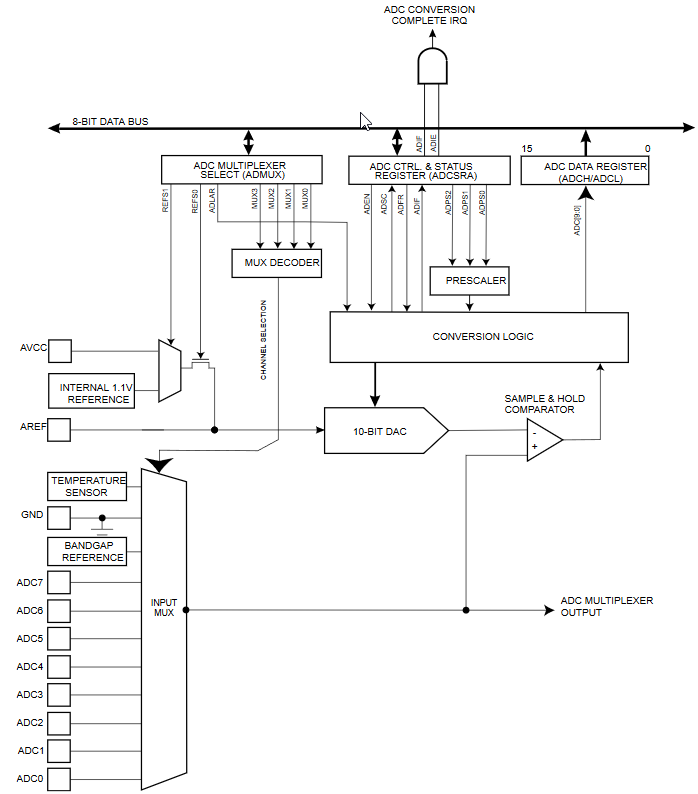# A/D converter - Measurement with an interrupt when done

Zápisník experimentátora

Hierarchy:

In the previous article, we have programmed an analog measurement that did not block Arduino during the measurement. The end of the measurement was checked using the bit `ADSC`. The end of analog measurement can also be controlled more conveniently with the interrupt that Arduino calls at the end of the measurement. In this article, we'll show you how to program it.## Example

If we use variables in the normal code also in interruptions, we need to label them as `volatile` so that the compiler knows they should update them for each use and write them to RAM. The registry setting is similar to the previous example, only the bit `ADIE` has been added to enable the use of the interrupt at the end of the A/D conversion.

The rest of the program consists of two conditions that handle the end of the A/D conversion, output the result to the serial port, and start the next analog conversion.

``````const byte adc_pin = A0; // = 14 (pins_arduino.h)
unsigned int something_different = 0;

void setup() {
Serial.begin (115200);

| ((adc_pin - 14) & 0x07); // Arduino Uno to ADC pin
}

}

void loop() {
Serial.print(", something_different=");
Serial.println(something_different);
delay(500);
}

// Start new conversion
// start the conversion
}

// Do something
something_different++;
}``````

## Source code

The source code is located on the GitHub server.

08.10.2018